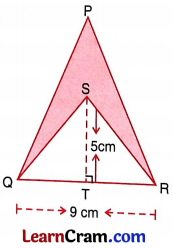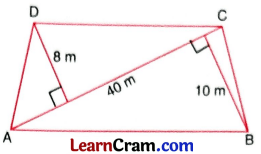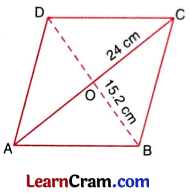# DAV Class 7 Maths Chapter 11 Worksheet 4 Solutions

The DAV Maths Class 7 Solutions and DAV Class 7 Maths Chapter 11 Worksheet 4 Solutions of Perimeter and Area offer comprehensive answers to textbook questions.

## DAV Class 7 Maths Ch 11 WS 4 Solutions

Question 1.
The area of a triangle is 90 cm2. If its base is 15 cm, find its altitude.
Area of triangle = $$\frac{1}{2}$$ × base × altitude
90 = $$\frac{1}{2}$$ × 15 × altitude
Altitude = $$\frac{90 \times 2}{15}$$ = 12 cm
Hence the required altitude = 12 cm.

Question 2.
Find the base of a triangle whose area is 0.48 dm2 and altitude is 8 cm.
Area = 0.48 dm2
= 0.48 x 100 cm2 = 48 cm2
Area of triangle = $$\frac{1}{2}$$ × base × altitude
⇒ 48 = $$\frac{1}{2}$$ × base × 8
⇒ 48 = base × 4
base = $$\frac{48}{4}$$ = 12 cm
Hence the required base = 12 cm.

Question 3.
Find the area of a right triangle whose hypotenuse is 13 cm and one side is 5 cm.
Let the other side be x cm From Pythagorous theorem,
x2 + (5)2 = (13)2
x2 + 25 = 169
x2 = 169 – 25 = 144
x = 12 cm
Area = $$\frac{1}{2}$$ × base × altitude
= $$\frac{1}{2}$$ × 12 × 5
= 30 cm2
Hence the required area = 30 cm2.Question 4.
Find the area of an isosceles right triangle whose equal sides are 15 cm each.
Here base = height = 15 cm
Area of the triangle = $$\frac{1}{2}$$ × base × height
= $$\frac{1}{2}$$ × 15 × 15
= $$\frac{225}{2}$$
= 112.5 cm2
Hence the required area = 112.5 cm2.

Question 5.
Find the area of an isosceles right triangle having the length of each equal sides 5 cm.
Here base = height = 5 cm
∴ Area = $$\frac{1}{2}$$ × base × height
= $$\frac{1}{2}$$ × 5 × 5 = $$\frac{25}{2}$$ = 12.5 cm2
Hence the required area = 12.5 cm2.

Question 6.
The base of a triangular field is three times its height. If the cost of cultivating the field at ₹ 36 per hectare is ₹ 486, find its base and height (1 hectare = 10,000 m2).
Area of the triangular field = $$\frac{486}{36}=\frac{27}{2}$$ hectare
= $$\frac{1}{2}$$ × 10000 m2
= 27 × 5000 m2
= 135000 m2

Let the height be x m
∴ Base = 3 x m
Area = $$\frac{1}{2}$$ × base × height
135000 = $$\frac{1}{2}$$ × 3x × x
135000 × 2 = 3x2
270000 = 3x2
x2 = $$\frac{270000}{3}$$
x2 = 90000
∴ x = 300
Hence the height is 300 m and base = 3 × 300
= 900 m.

Question 7.
In the given figure, ST = 5 cm, QR = 9 cm. The area of the larger triangle is 50 cm2 . What is the area of shaded region?Area of the smaller Δ SQT
= $$\frac{1}{2}$$ × QR × ST
= $$\frac{1}{2}$$ × 9 × 5
= $$\frac{45}{2}$$ = 22.5 cm
Area of the bigger ∆ PQR = 50 cm2
Area of the shaded region = 50 cm2 – 22.5 cm2 = 27.5 cm2
Hence the required area = 27.5 cm2 .

Question 8.
The area of a rhombus is 98 m2 . If one of its diagonal is 14 m, find the other diagonal
If d1 and d2 be the diagonals of the rhombus, then
Area of the rhombus = $$\frac{1}{2}$$ × d1 × d2
98 = $$\frac{1}{2}$$ × 14 × d2
$$\frac{98 \times 2}{14}$$ = d2
d2 = 14 cm
Hence the length of the required diagonal = 14 cm.

Question 9.
The diagonals of a rhombus are 8 cm and 6 cm. Find its area.
Area of a rhombus = $$\frac{1}{2}$$ × d1 × d2
= $$\frac{1}{2}$$ × 8 × 6 = 4 × 6 = 24 cm2
Hence the required area = 24 cm2.Question 10.
Find the area of a rhombus whose side is 13 cm and altitude is 2 cm.
Area of a rhombus = Base × Altitude
= 13 × 2 = 26 cm2
Hence the required area = 26 cm2.

Question 11.
The area of a rhombus is 220.5 cm2. If its altitude is 17.5 cm, find the length of each side of the rhombus.
Area of rhombus = Base × Altitude
220.5 = Base × 17.5
Hence the side of the rhombus = 12.5 cm.

Question 12.
A diagonal of a quadrilateral is 40 m long and the perpendiculars to it from the opposite corner are 8 m and 10 m respectively. Find its area.Area of □ ABCD = area of Δ ABC + area of Δ ADC
= $$\frac{1}{2}$$ × AC × BE + y × AC × DF
= $$\frac{1}{2}$$ × AC × (BE + DE)
= $$\frac{1}{2}$$ × 40 × (8 + 10)
= $$\frac{1}{2}$$ × 40 × 18
= 20 × 18 = 360 m2
Hence the required area = 360 m2.

Question 13.
Find the area of a rhombus whose side is 15 cm long while one of the diagonal is 24 cm long.Join BD
As the diagonals of a rhombus bisect each other at right angle,
∴ OA = $$\frac{24}{2}$$ = 12 cm
In right ΔAOD
OA2 + OD2 = AD2 (By Pythagoras Theorem)
(12)2 + OD2 = (15)2
144 + OD2 = 225
OD2 = 225 – 144
OD2 = 81
OD = 9 cm
BD = 9 × 2 = 18 cm
Area of the rhombus = $$\frac{1}{2}$$ × d1 × d2
= $$\frac{1}{2}$$ × 24 × 18
= 12 × 18
= 216 cm2
Hence the area of the rhombus = 216 cm2.